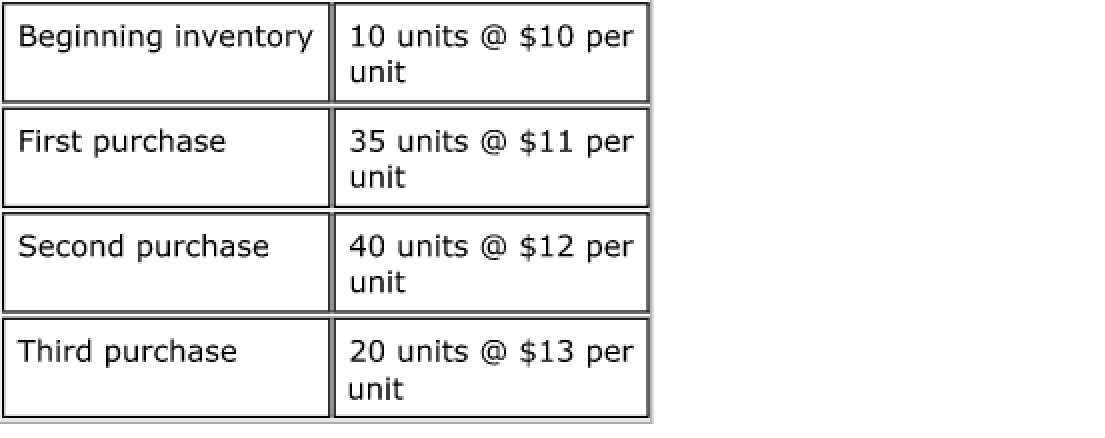# Problem: Consider the following: There were 105 units available at a total cost of $1,225. Eighty-three units were sold. Use the LIFO method of inventory costing and determine the cost of goods sold. a.$993 b. $968 c.$962 d. $941 ###### FREE Expert Solution ###### Problem Details Consider the following: There were 105 units available at a total cost of$1,225. Eighty-three units were sold. Use the LIFO method of inventory costing and determine the cost of goods sold.

a. $993 b.$968

c. $962 d.$941Frequently Asked Questions

What scientific concept do you need to know in order to solve this problem?

Our tutors have indicated that to solve this problem you will need to apply the Periodic Inventory - FIFO, LIFO, and Average Cost concept. You can view video lessons to learn Periodic Inventory - FIFO, LIFO, and Average Cost. Or if you need more Periodic Inventory - FIFO, LIFO, and Average Cost practice, you can also practice Periodic Inventory - FIFO, LIFO, and Average Cost practice problems.

What is the difficulty of this problem?

Our tutors rated the difficulty ofConsider the following: There were 105 units available at a...as medium difficulty.